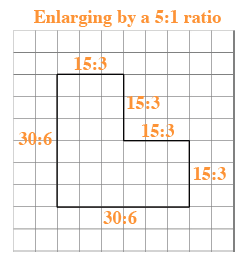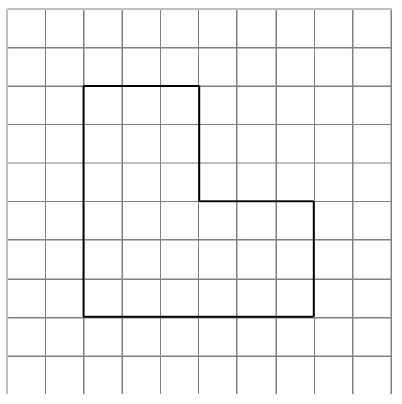### Home > CC1 > Chapter 6 > Lesson 6.2.5 > Problem6-117

6-117.

Copy the diagram at right on your own graph paper. Then enlarge or reduce it by each of the following ratios.

If we use each ratio as new/original, then the example below shows the lengths of a new diagram that would have sides that are five times as long as the original.1.  $\frac { 3 } { 1 }$

If you choose to use a ratio of new/original, you will need to enlarge the figure. Use the example.
Can you draw your own diagram?

2. $\frac { 2 } { 3 }$

$\text{For part (b), the new diagram will be }\frac{2}{3}\text{ the size of the original.}$